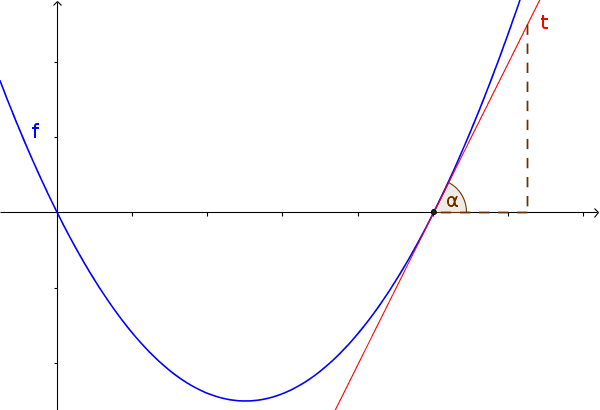Math Derivative applications Slope angle

# Slope angle

With the derivation you can also determine the slope angle at a point $x$.!

### Remember

The slope angel $\alpha$ of a function $f$ at the point $x$ is:
$\alpha=\arctan(f'(x))$

### Example

Calculate the slope angle of the function $f(x)=x^2$ at $x=1$.

Antiderivative: $f(x)=x^2$
Derivative: $f'(x)=2x$

Insert:
$\alpha=\arctan(f'(x))$
$\alpha=\arctan(f'(1))$
$f'(1)=2\cdot1=2$
$\alpha=\arctan(2)\approx63,43°$
i

### Hint

Frequently, calculators use $\arctan$ instead of $\tan^{-1}$. Both methods are the same.